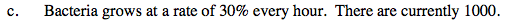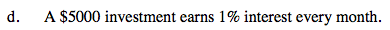### Home > PC > Chapter 8 > Lesson 8.1.3 > Problem8-46

8-46.
1. Recall that an exponential function, with the x-axis as an asymptote, can be expressed as y = a · mx where a is the initial value and m is the multiplier. Find the initial value, the multiplier, and the time units in each of the following situations. Homework Help ✎

1. Bread costs $1.87 now and is increasing in price by 2% each month. 2. A car is worth$12000 now and is depreciating in value by 12% each year.

3. Bacteria grows at a rate of 30% every hour. There are currently 1000.

4. A $5000 investment earns 1% interest every month.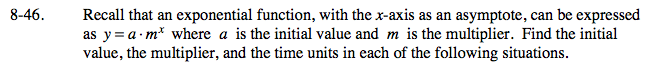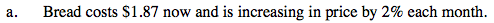Initial value =$1.87, multiplier = 1 + 0.02 = 1.02 , time is in months.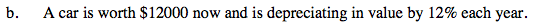Multiplier will be down 0.12 from 1.0.